Chapter 18. Chemical Thermodynamics

# Gibbs Free Energy

Jessie A. Key

Learning Objectives

• To gain an understanding of Gibbs free energy.
• To understand the relationship between the sign of Gibbs free energy change and the spontaneity of a process.
• To be able to determine Gibbs free energy using standard free energies of formation.

J. Willard Gibbs (1839–1903) proposed a single state function to determine spontaneity: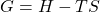where H is the enthalpy of the system, S is the entropy of the system, and G is Gibbs free energy.

The change in Gibbs free energy, ΔG, is the maximum amount of free energy available to do useful work. For an isothermal process, it can be expressed as: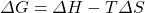or at standard conditions: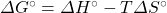This single term, Gibbs free energy (G), allows us to avoid calculating the entropy of the surroundings. It is really just a simplification of our previous method of estimating spontaneity: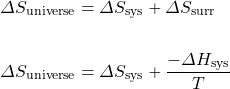Multiply both sides of the equation by −T: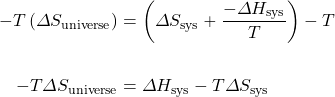Therefore,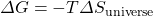.

As a result of this relationship, the sign of Gibbs free energy provides information on the spontaneity of a given reaction: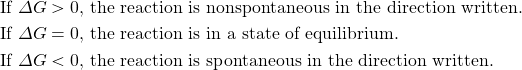The significance of the sign of a change in Gibbs free energy parallels the relationship of terms from the equilibrium chapter: the reaction quotient, Q, and the equilibrium constant, K.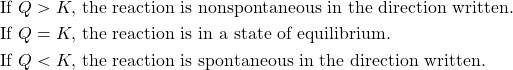Example 18.4

Calculate ΔG° for a reaction where ΔH° is equal to 36.2 kJ and ΔS° is equal to 123 J/K at 298 K. Is this a spontaneous reaction?

Solution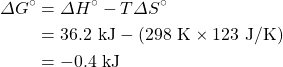Therefore the reaction is spontaneous because ΔG° is negative.

# Determining ΔG° from Standard Free Energy of Formation

The standard Gibbs free energy change, ΔG°, for a reaction can be calculated from the standard free energies of formation, ΔG°f .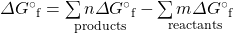where n and m are the coefficients in the balanced chemical equation of the reaction.

Standard free energies of formation values are listed in the appendix, “Standard Thermodynamic Quantities for Chemical Substances at 25°C.”

Example 18.5

Calculate the standard free energy change for the following reaction, using standard free energies of formation:

5C(s) + 2SO2(g) → CS2(g) + 4CO(g)

Is this a spontaneous reaction?

Solution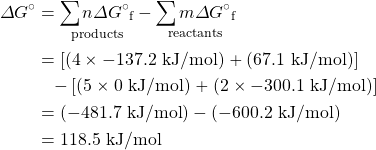ΔG° has a positive value, so this is not a spontaneous process.

Key Takeaways

• The change in Gibbs free energy (ΔG) is the maximum amount of free energy available to do useful work.
• If Δ> 0, the reaction is nonspontaneous in the direction written. If Δ= 0, the reaction is in a state of equilibrium. If Δ< 0, the reaction is spontaneous in the direction written.
• The standard Gibbs free energy change, ΔG°, for a reaction can be calculated from the standard free energies of formation, ΔG°f.